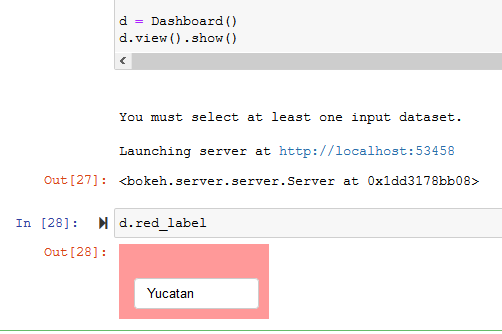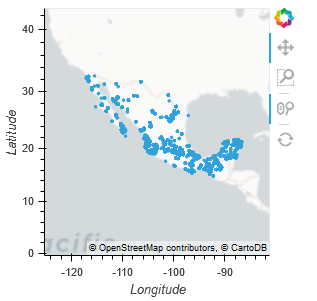# Why do some Panel elements dissappear while others persist?

I’ve made an app which so far works very well. The user can configure some input file settings, draw polygons on the map, and the drawn polygons are used as geographic extents to retrieve intersecting points from the user’s input file(s). It looks like this.

At the bottom you can see some colour text inputs which are `param.String()` wrapped in `panel.Row`. I want these to remain permanently on the page, however, when the function triggered by the `Run` button is called the coloured text boxes disappear while the remaining elements remain. After pushing `Run` the app looks like this.

Can someone explain to me why those text boxes are being removed, and how to prevent them from being removed? The objects persist in the Python kernel and their state is preserved; I can go to the Jupyter Notebook and do this to see that the object exists and the text value I entered persists:The following code is a working example which demonstrates the issue when run in a Jupyter Notebook.

…it’s a big blob of code, for which I apologize, however, most responses on this forum begin with “Please post a working example” so I figured I’d start with that. Thanks in advance to anyone who takes a look.

Running it will require a sample input file be placed in a folder called `sample_data\` in the same directory as the notebook. This file can be used for testing: ebird_10000.csv (1.5 MB)

``````import panel as pn
import param
import geoviews as gv
import holoviews as hv
import pandas as pd
import geopandas as gpd
from shapely.geometry import Point, Polygon
from cartopy import crs

gv.extension('bokeh',logo=False)
pn.extension()

class Inputs(param.Parameterized):

input_file = param.FileSelector(path='.\sample_data\*.csv', doc='A .csv file selector for input data')
x_field    = param.Selector(doc='A field selector for longitude')
y_field    = param.Selector(doc='A field selector for latitude')
id_field   = param.Selector(doc='A field selector for the identifier')
data       = None

@param.depends('input_file', watch=True)
def update_inputs(self):

numeric_cols                = list(df.select_dtypes(include=['float64']).columns)
columns                     = [x for i,x in enumerate(df.columns) if x !='Unnamed: 0']
self.param.x_field.objects  = numeric_cols
self.x_field                = numeric_cols
self.param.y_field.objects  = numeric_cols
self.x_field                = numeric_cols
self.param.id_field.objects = columns
self.id_field               = columns
self.data                   = df

class Mapview(param.Parameterized):

tiles         = gv.tile_sources.CartoEco()
aoi_colours   = ['red','blue','green','orange','purple']
aoi_stream    = hv.streams.PolyDraw(source=aoi_polygons, num_objects=5,styles={'fill_color': aoi_colours})
template_df   = pd.DataFrame({'lng': [], 'lat': []}, columns=['lng', 'lat'])
dfstream      = hv.streams.Buffer(template_df, index=False, length=10000, following=False)
points        = hv.DynamicMap(gv.Points, streams=[dfstream])
map_layout    = tiles * aoi_polygons * points

def show_map(self):
# set style options on map_layout
self.map_layout.opts(
# the ratio of WMTS height:width must be eqaul to the data_aspect value (0.5)
# or the map will stretch/skew
gv.opts.WMTS(global_extent=True,width=1200,height=600,show_grid=False,xaxis=None,yaxis=None),
gv.opts.Polygons(fill_alpha=0.1),
gv.opts.Points(size=5, color='green', fill_alpha=0.3, line_alpha=0.4)
)
return self.map_layout.opts(data_aspect=0.5)

class Aoi(param.Parameterized):
aoi = param.String('Name')

def set_aoi_textbox_style(self, row, color='rgba(0,0,0,0)'):
row.background = color
row.objects.value = ''
row.objects.name = ''
row.objects.width = 145

class Dashboard(param.Parameterized):

input1    = Inputs()
input2    = Inputs()
input3    = Inputs()
input4    = Inputs()
input5    = Inputs()
mapview   = Mapview()

run_button = param.Action(lambda x: x.param.trigger('run_button'), label='Run')

# create colour-coded text input boxes to allow the user to optionally provide names for
# the AOIs drawn on the map

aois = []

red = Aoi()
red_label = pn.Row(red, width=150)
red.set_aoi_textbox_style(red_label, 'rgba(255,0,0,0.4)')
aois.append(red_label)

blue = Aoi()
blue_label = pn.Row(blue, width=150)
blue.set_aoi_textbox_style(blue_label, 'rgba(0,0,255,0.4)')
aois.append(blue_label)

green = Aoi()
green_label = pn.Row(green, width=150)
green.set_aoi_textbox_style(green_label, 'rgba(0,255,0,0.4)')
aois.append(green_label)

orange = Aoi()
orange_label = pn.Row(orange, width=150)
orange.set_aoi_textbox_style(orange_label, 'rgba(255,165,0,0.4)')
aois.append(orange_label)

purple = Aoi()
purple_label = pn.Row(purple, width=150)
purple.set_aoi_textbox_style(purple_label, 'rgba(150,0,150,0.4)')
aois.append(purple_label)

def show_aoi_gui(self):

return pn.Row(pn.Spacer(width=5),
self.red_label,pn.Spacer(width=3),
self.blue_label,pn.Spacer(width=3),
self.green_label,pn.Spacer(width=3),
self.orange_label,pn.Spacer(width=3),
self.purple_label,pn.Spacer(width=3))

"""While Geoviews will automatically project coordinates in degrees into meters to
match the coordinate system of the WMTS object, the Geopandas.GeoDataFrame.intersects()
method employed in the run_analysis() function requires the point coordinates to have
the same coordinates as the polygon geometries. Therefor the point coordinates must be projected
to web mercator (epsg:3857)"""
def project_to_meters(self, df, x, y):
try:
df.loc[:,'x_meters'], df.loc[:,'y_meters'] = lnglat_to_meters(df[x],df[y])
geometry = [Point(xy) for xy in zip(df.x_meters, df.y_meters)]
web_merc = {'init': 'epsg:3857'}
return gpd.GeoDataFrame(df, crs=web_merc, geometry=geometry)
except:
print("""Could not project specified coordinate fields to meters. Make sure the X Field and Y Field
values have been set correctly.\n""")

@param.depends('run_button')
def run_analysis(self):
try:
# clear previous results
self.mapview.dfstream.clear()

# Convert each input into a GeoDataFrame and project coordinates to meters
datasets  = []
id_fields = []
for i in [self.input1, self.input2, self.input3, self.input4, self.input5]:
if isinstance(i.data, pd.core.frame.DataFrame):
gdf = self.project_to_meters(i.data, i.x_field, i.y_field)
datasets.append(gdf)
id_fields.append(i.id_field)

if len(datasets)>0:
# Convert polygons drawn on the map into Shapely polygon geometries
poly_data = d.mapview.aoi_stream.data
polygons = []
for r in range(len(poly_data['xs'])):
xs = poly_data['xs'][r]
ys = poly_data['ys'][r]
vertices = list(zip(xs,ys))
polygons.append(Polygon(vertices))

# Do intersections of each polygon on each GeoDataFrame & send results to the map
df_all_polys = pd.DataFrame()
df_final = pd.DataFrame()
poly_labels = []

for i in range(len(datasets)):
for p in polygons:
# if the user does not specify a name for the aoi, use
# the colour as the name
if (self.aois[polygons.index(p)].objects.value == 'Name'):
poly_label = self.mapview.aoi_colours[polygons.index(p)] + '_aoi'
# else use the name provided by the user
else:
poly_label = self.aois[polygons.index(p)].objects.value + '_user_provided'
poly_labels.append(poly_label)
datasets[i][poly_label] = datasets[i].intersects(p)
selected = datasets[i].loc[datasets[i][poly_label]==True].drop(columns='geometry')
self.mapview.dfstream.send(pd.DataFrame(selected[['lng','lat']]))
selected.rename(columns={id_fields[i]:'identifier'},inplace=True)
df_all_polys = pd.concat([df_all_polys,selected[['identifier',poly_label]]],axis=0,ignore_index=True)

for ident in pd.unique(df_all_polys['identifier']):
dfx = df_all_polys.loc[df_all_polys['identifier']==ident]
poly_values = {'identifier':[ident]}
for label in poly_labels:
# returns true if any of the values are true
poly_values[label] = [dfx[label].any()]
df_ident = pd.DataFrame.from_dict(poly_values)
df_final = pd.concat([df_final, df_ident],axis=0)

def summarize_polys(row):
cols = list(row.axes)
s = []
for c in cols:
if '_aoi' in c:
if row.values[cols.index(c)]:
s.append(c.split('_aoi'))
if '_user_provided' in c:
if row.values[cols.index(c)]:
s.append(c.split('_user_provided'))
return ','.join(s)

summary = df_final.copy()

try:
summary['Found_In'] = summary.apply(lambda row: summarize_polys(row),axis=1)
# remove the '_user_provided' flag from column names where it exists
for col in summary.columns.to_list():
if '_user_provided' in col:
summary.rename(columns={col : col.split('_user_provided')}, inplace=True)

return pn.widgets.DataFrame(summary, disabled=True, fit_columns=True, width=700)

except:
print('There was an error creating the final output summary table.\n')

else:
print('You must select at least one input dataset.\n')

except:
print('Something went wrong in the run_analysis() function.\n')
# show the message in the Table View tab of the dashboard
return('Something went wrong in the run_analysis() function.')

def view(self):

desc = """This is a demonstration dashboard with incomplete functionality. Its purpose
is to sit here and look pretty. We can put graphics and stuff in here to
make it look all fancy."""

logo = '.\images\logo_panel_stacked_s.png'

button_desc = """The <i>Run</i> button will execute the spatial intersection and generate
a table of identifiers found within each polygon and in multiple polygons.<br><br>
Push the <i>Run</i> button after configuring all inputs and drawing polygons on the map."""

return pn.Row(
pn.Column(
'## Description',
desc,logo),
pn.Column(
'### Configure Inputs',
pn.Tabs(
('Input 1',self.input1),
('Input 2',self.input2),
('Input 3',self.input3),
('Input 4',self.input4),
('Input 5',self.input5)),
button_desc,
self.param['run_button']),
pn.Column(pn.Tabs(
('Map View', self.mapview.show_map),
('Results Table', self.run_analysis)),
pn.Column(
pn.Row(pn.Spacer(width=5),'Optionally provide names for drawn AOIs:'),
self.show_aoi_gui
)))

d = Dashboard()
d.view().show()`````````

I tried to take a look. But I cannot get it working.

### Steps

1. Create a new conda environment and activate it
2. `conda install panel param geoviews holoviews pandas datashader shapely cartopy`
3. Navigate to the folder where you have the file with the above code
4. create the `sample_data` and put two copies of the ebird_1000.csv data in that folder. One won’t work as that will now allow you to select a dataset.
5. Run the file: `python discourse_1260.py` (or similar name)
6. Navigate to http://localhost:64001/ (or similar)
7. Select `ebird_1000.csv`, x_field: lng, y_fild: lat, id_field: comName
8. Activate the Polygon drawing Tool
9. Draw some polygons.
10. Click Run

It looks like

But nothing happens except I get a warning

``````C:\Users\USERNAME\AppData\Local\Continuum\anaconda3\envs\geo\lib\site-packages\pyproj\crs\crs.py:53: FutureWarning: '+init=<authority>:<code>' syntax is deprecated. '<authority>:<code>' is the preferred initialization method. When making the change, be mindful of axis order changes: https://pyproj4.github.io/pyproj/stable/gotchas.html#axis-order-changes-in-proj-6
return _prepare_from_string(" ".join(pjargs))
``````

Hi @Marc, thanks for taking a look.

I neglected to mention that my sample data is located only in Mexico; if you draw polygons over Mexico it should work. That `ebird_10000.csv` file plots like so:create the `sample_data` and put two copies of the ebird_1000.csv data in that folder. One won’t work as that will now allow you to select a dataset.

I have encountered this behaviour as well. The `param.FileSelector` seems to use the first file it finds as it’s default value but then that file is not selectable. I’m not sure how to handle that. I would expect `param.FileSelector` to allow us to select any of the files in a given directory. My assumption is that I’ve not implemented it correctly.

This is strange. When I use a smaller input file that has only 10 rows the dashboard works as I expect and the coloured text inputs at the bottom do not disappear.

Here is the smaller file I used: ebird_10.csv (1.5 KB)

I’ve just noticed that the coloured text boxes are not actually disappearing, they’re just being pushed down the page in proportion to the size of the input file I select. When I use an input file with 100 rows it looks like this; the text boxes are pushed down as indicated by the arrows.

With a 200 row input it does this:

So it seems I misidentified the problem. The GUI elements are not vanishing, they are being pushed down the page.

I don’t understand why that is happening though. The class method I wrote to make the layout does not seem contain anything that would cause the issue. But clearly something in mode code is pushing empty space into the layout.

I’ve discovered what’s happening. The map is one of two Panel elements contained in a `pn.Tabs`, the other being a results table. The issue has nothing to do with the input file, it has to do with the number of rows in the output table. When I switch to the Results Tab and scroll all the way down to the bottom I can see that it’s the results table that is pushing the text boxes down. Now I just need to figure out what to do about this.

1 Like

For now I have resolved the problem by changing the layout. This works fine.

So the issue was my own design, nothing to do with Panel. I might look into limiting the size of the output dataframe and having a scrollbar or something like that.

3 Likes

Great that you solved it.

For me it seams like a bug though. A user would next expect this behaviour. Feel free to post a bug with a small reproducible example.

Alternative solutions could be to set the Tabs to only load the visible tab, add scrollbars to the table on the 2nd panel, set a max height on the tabs or table. (I believe).

I don’t think there’s a bug. I was originally setting the `width` of my `pn.widgets.DataFrame()` but not the `height`. Setting the `height` and leaving the `height_policy` at the default value of `auto` works; when the height of the dataframe overflows the explicitly set `height` a scrollbar appears and my other GUI components do not get pushed around.

So setting `height=500`

``````pn.widgets.DataFrame(summary, disabled=True, fit_columns=True, width=700, height=500)
``````

…yields this, which works very well.

2 Likes